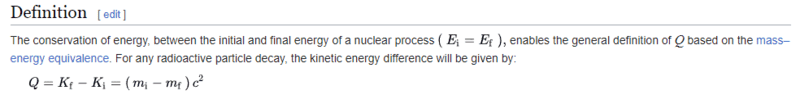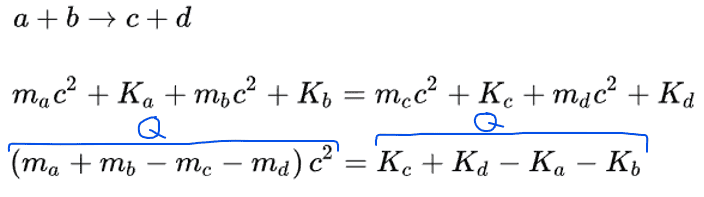# Q value for a radioactive decay

• I
• eneacasucci

#### eneacasucci

The Q value for a reaction is the amount of energy absorbed or released during the nuclear reaction. Is it correct to say that for a radioactive decay, since it is a spontaneous process, to occur the Q-value must be Q>0?

Last edited:

If Q < 0 where does the energy come from?

••eneacasucci and vanhees71
You can post a negatiove emoji if you want. Maybe then someone will hand you your answer on a silver platter. Or you could think about it and maybe learn something.

•weirdoguy
You can post a negatiove emoji if you want. Maybe then someone will hand you your answer on a silver platter. Or you could think about it and maybe learn something.

1) I didn't use a negative emoji, I used the reaction "wow", is it a negative emoji? I'm new here so I don't know if using that emoji, I caused you some problem. The reaction was "wow" because it expressed the sense of astonishment for thinking about where the energy could come from if Q<0

2) From the tone of your reply I infer you are somehow offended or annoyed, that was not my intention. For my part I don't think I deserve this treatment, I don't seem to have demanded an answer on a silver platter, on the contrary, I had thought about the question and had already posed an answer to it, as can be seen from my post what I was asking for was only a confirmation of my reasoning, I don't think I was arrogant or presumptuous, nor did I demand a private quantum physics lesson.

3) I am new to this forum, as I have already said, but I don't think anyone here is obliged to answer or not answer questions and topics posed by users. I thank you sincerely for your time and reply but you were not obliged to do so, please don't make it look like you were forced by me.

4) If I'm on a physics forum I think it is of course because I want to learn something. I have been in hospital for 4 months and I wasn't able to keep up with the lectures of university, I asked my professor some questions but he never replied to me so I decided to ask my questions here.

Last edited by a moderator:
••berkeman, weirdoguy and malawi_glenn
The first thing to remember is that in Nature everything can happen, if it's not forbidden by a conservation law. Thus if you think about a reaction (be it in nuclear or particle physics or also chemistry) first start to think about the conservation laws.

First of all there is energy and momentum. Now consider your nucleus, and let's assume we look at a simple decay like ##\alpha## decay, where we have a nucleus, ##A_1##, decaying to a nucleus ##A_2## and an ##\alpha## particle. Since in nuclear physics the binding energies are not too well negligible against the masses of the particles in question, we have to do the calculation relativistically.

In such cases energy and momentum conservation is nicely combined to the conservation of the four-momentum vector. For the initial nucleus we can assume that we choose a reference frame, where it is at rest, which makes the calculation as simple as possible. The four-momentum of this initial nucleus is
$$p_1=\begin{pmatrix}m_1 c \\\vec{0} \end{pmatrix}.$$
Note that the time-component ##p^0## of the four-momentum of a particle is always ##p^0=E/c##, where ##E## is the energy of the particle including the rest energy ##E_0=m c^2##, where ##m## is the (invariant!) mass of the particle. Each four-momentum also obeys the "on-shell condition",
$$p \cdot p=p^2=(p^0)^2-\vec{p}^2=m^2 c^2.$$
In the final state we have the four-momentum of the ##\alpha## particle and the daughter nucleus,
$$p_{\alpha}=\begin{pmatrix}E_{\alpha}/c \\ \vec{p}_{\alpha} \end{pmatrix}, \quad p_2=\begin{pmatrix} E_2/c \\ \vec{p}_2 \end{pmatrix}.$$
Then because of energy and momentum conservation
$$p_{\alpha}+p_2=p_1.$$
Form this we immideately get
$$\vec{p}_2=-\vec{p}_{\alpha}=:\vec{p},$$
i.e.,
$$p_{\alpha}=\begin{pmatrix} E_{\alpha}/c,-\vec{p} \end{pmatrix}, \quad p_2=\begin{pmatrix} E_2/c \\ \vec{p} \end{pmatrix}.$$
From the on-shell conditions you get
$$E_{\alpha}/c=\sqrt{m_{\alpha}^2 c^2+\vec{p}^2}, \quad E_2/c=\sqrt{m_2^2 c^2+\vec{p}^2}.$$
From the energy conservation equation you have
$$m_1 c=\sqrt{m_{\alpha}^2 c^2+\vec{p}^2} + \sqrt{m_2^2 c^2+\vec{p}^2}.$$
After some algebra you get
$$\vec{p}^2=\frac{c^2}{4 m_1^2}(m_1-m_2-m_{\alpha})(m_2-m_2+m_{\alpha})(m_1+m_2-m_{\alpha})(m_1+m_2+m_{\alpha}).$$
Since this must be ##> 0## you must have ##m_1>m_2+m_{\alpha}## or ##Q=m_1-(m_2+m_{\alpha})>0## [EDIT: typo corrected in view of #6].

Last edited:
••Astronuc and eneacasucci
Since this must be ##> 0## you must have ##m_1>m_2+m_{\alpha}## or ##Q=m_1-(m_2+m_{\alpha})<0##.
Thank you so much for the detailed answer and for your time.
I hope I can live up to the debate.
It all makes sense to me except the very last thing:
I'm not able to understand why from this ##m_1>m_2+m_{\alpha}## (that is correct to me) you went to this ##Q=m_1-(m_2+m_{\alpha})<0##
I do not understand why taking the terms to the left of the inequation has changed the direction of the inequation

For me it is: ##m_1>m_2+m_{\alpha} \rightarrow Q=m_1-(m_2+m_{\alpha})>0## just taking the terms of the inequation to the left
Am I wrong?

P.S. I can't understand what's wrong with my LaTeX :(

Last edited:
That's of course a typo, which I've corrected.

Your LaTeX seems to spoilded by using some strange symbol for the > sign. Here it works just fine:

##m_1>m_2+m_{\alpha} \rightarrow Q=m_1-(m_2+m_{\alpha})>0##

•eneacasucci
That's of course a typo, which I've corrected.

Your LaTeX seems to spoilded by using some strange symbol for the > sign. Here it works just fine:

##m_1>m_2+m_{\alpha} \rightarrow Q=m_1-(m_2+m_{\alpha})>0##
Thank you so much, so we have shown that for the alpha decay Q>0

I've just one last question:
From the conservation of energy, we see that the Q-value can be expressed also as the difference between the kinetic energy (K) of the final particles and the initial one ##Q=K_2+K_{\alpha}-K_1##, if we choose a reference frame where A1 is at rest we get ##K_1=0## and ##Q=K_2+K_{\alpha}>0## since the kinetic energy can't be negative.
I suppose that if we change the reference system, for example considering the reference system for which the second nucleus A2 is at rest, the Q value should not change but it is simply less immediate to establish its sign, right?
If the Q value is >0 for one reference system, can I say it is for any reference system I choose (It should not be a quantity that depends on the chosen reference system)?

That's in general not accurate enough for nuclear physics, because you have to take into account that the binding energies are a few MeV per nucleon, which is not negligible with the nucleon mass of around 938 MeV, i.e., the Q-value is defined aas ##Q=E_2+E_{\alpha}-E_1##, where the ##E##'s are the energies including the rest mass energies, i.e., ##E=c \sqrt{m^2 c^2+\vec{p}^2}##.

That's in general not accurate enough for nuclear physics, because you have to take into account that the binding energies are a few MeV per nucleon, which is not negligible with the nucleon mass of around 938 MeV, i.e., the Q-value is defined aas ##Q=E_2+E_{\alpha}-E_1##, where the ##E##'s are the energies including the rest mass energies, i.e., ##E=c \sqrt{m^2 c^2+\vec{p}^2}##.
I was referring to this definition:(https://en.wikipedia.org/wiki/Q_value_(nuclear_science))
and I thought it was general since it comes from the conservation of energy that of course takes into account the kinetic energy and the rest mass energy.

i.e.•eneacasucci
There are also kinematical constraints from energy-momentum conservation + on-shell conditions. For details see

https://pdg.lbl.gov/2022/reviews/rpp2022-rev-kinematics.pdf
Thank you! I study engineering so we didn't go that deep in the physics but I'm interested in it.
Is it a section of a book or something else that I could buy? I see this is chapter 49, I wish I could study also the others to have a whole insight.

•vanhees71
I see this is chapter 49, I wish I could study also the others to have a whole insight.
PDG is not a textbook per se, it is a collection of experimental results for subatomic particle properties. It does contain breif introductory/summary chapters.

Any introductory nuclear physics textbook should suffice.

•vanhees71 and eneacasucci
The "Review of Particle Physics", being updated every 2 years, is completely free of charge. It's most convenient to use the website to look things like particle properties up:

https://pdg.lbl.gov/

You can also download the complete print version from here. It's open access

•